Next: About this document ... Up: lab_template Previous: lab_template

Subsections

# Taylor Polynomials

## Purpose

The purpose of this lab is to use Maple to introduce you to Taylor polynomial approximations to functions, including some applications.

## Background

The idea of the Taylor polynomial approximation of order
at, written
, to a smooth function
is to require thatandhave the same value at. Furthermore, their derivatives atmust match up to order. For example the Taylor polynomial of order three forat
would have to satisfy the conditions
You should check for yourself that the cubic polynomial satisfying these four conditions is
The general form of the Taylor polynomial approximation of ordertois given by the following

Theorem 1   Suppose thatis a smooth function in some open interval containing. Then theth degree Taylor polynomial of the functionat the pointis given by
We will be seeing this formula a lot, so it would be good for you to memorize it now! The notation
is used in the definition to stand for the value of the-th derivative of
at. That is,,
, and so on. By convention,
. Note that
is fixed and so the derivativesare just numbers. That is, a Taylor polynomial has the form
which you should recognize as a power series that has been truncated.

## Accuracy and Tolerance

To measure how well a Taylor Polynomial approximates the function over a specified interval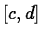, we define the tolerance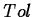ofto be the maximum of the absolute error
over the interval.

## Maple Commands

To use the Taylor and TayPlot commands you need to load the CalcP7 package.
>with(CalcP7);


The exponential function can be approximated at a base point zero with a polynomial of order four using the following command.

>Taylor(exp(x),x=0,4);

You might want to experiment with changing the order. To seeand its fourth order polynomial use
>TayPlot(exp(x),x=0,{4},x=-4..4);

This plots the exponantial and three approximating polynomials.
>TayPlot(exp(x),x=0,{2,3,4},x=-2..2);

Notice that the further away from the base point, the further the polynomial diverges from the function. the amount the polynomial diverges i.e. its error, is simply the difference of the function and the polynomial.
>plot(abs(exp(x)-Taylor(exp(x),x=0,3)),x=-2..2);

This plot shows that in the domain x from -2 to 2 the error around the base point is zero and the error is its greatest at x = 2 with a difference of over one. You can experiment with the polynomial orders to change the accuracy. If your work requires an error of no more than 0.2 within a given distance of the base point then you can plot your accuracy line y = 0.2 along with the difference of the function and the Taylor approximation polynomial.
>plot([0.2,abs(exp(x)-Taylor(exp(x),x=0,3))],x=-2..2,y=0..0.25);

We knew this would have some of its error well above 0.2. Change the order from three to four. As you can see there are still some values in the domain close to x = 2 whose error is above 0.2. Now try an order of 5. Is the error entirely under 0.2 between x = -2 and x = 2? Larger orders will work as well but order five is the minimum order that will keep the error under 0.2 within the given domain.

## Exercises

1. For the following functions and base points, determine what minimum order is required so that the Taylor polynomial approximates the function to within a tolerance of
over the given interval.
a)
, base point
, interval
.
b)
, base point, interval
.
c)
, base point
, interval.
d)
,base point
, interval
.

2. You read in the begining of the background that a Taylor Polynomial must have the same y-value, at the base point, as the original function. Also, the derivatives at that point must be the same. Three functions and three second-order polynomials are given; determine which function goes with which Taylor Polynomial by following the steps below.
a)
Enter the functions and the polynomials.
b)
Find the y-values of all six at.
c)
Find the first derivative at 0 of all six.
d)
Find the second derivative at 0 of all six.
e)
Which Taylor Polynomials go with which functions.Next: About this document ... Up: lab_template Previous: lab_template
Jane E Bouchard
2006-10-05